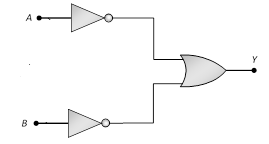# Physics Quiz on Digital Electronics for IIT JEE, NEET, Medical & Engineering Exam

0

## Physics Quiz on Digital Electronics

Physics Quiz on Digital Electronics: Previous trends reveal that NEET aspirants find Physics as the most challenging section amongst the other sections. But most of them fail to decipher the reason behind this. This particularly can be as the candidates taking up this examination are predominantly medical aspirants with their subject of interest being Biology. Also, sections like Physics are more on theories, laws, numerical as opposed to Biology which is more of fact-based, life sciences and comprising of substantial explanations. In this post we are providing you Physics quiz on Digital Electronics.

## Physics Quiz on Digital Electronics

Q1. The logic behind NOR gate is that it gives _______.
a) High output when both the inputs are low
b) High output when both the inputs are high
c) Low output when both the inputs are low
d) None of the above

a) High output when both the inputs are low

Q2. Which of the following logic gate is a universal gate?
a) OR
b) NOT
c) AND
d) NOR

d) NOR

Q3. The output of OR gate is 1, if ________.
a) If both inputs are zero
b) If either or both inputs are 1
c) If either input is zero
d) Only if both inputs are 1

b) If either or both inputs are 1

Q4. How many NAND gates are used to form an AND gate?
a) 1
b) 2
c) 3
d) 4

b) 2

Q5. Boolean Algebra is essentially based on _______.
a) Truth
b) Symbol
c) Logic
d) Numbers

c) Logic

Q6. The outputs of a NAND gate is 0, if _________.
a) both inputs are 0
b) both inputs are 1
c) one input is 0 and the other input is 1
d) both inputs are 1 or one of the inputs is 1 and the other one is 0

b) both inputs are 1

Q7. A gate in which all the inputs must be low to get a high output is ______.
a) NAND gate
b) NOR gate
c) Inverter
d) Both (b) and (c)

Q8. The following truth table corresponds to the which logic gate.

 Input Output A B X 0 0 0 0 1 1 1 1 1 1 0 1

a) NAND
b) OR
c) AND
d) XOR

b) OR

Q9. Which logic gate is represented by the following combination of logic gates?a) OR
b) NOR
c) AND
d) NAND

d) NAND

Q10. A logic gate is an electronic circuit which ________.
a) Makes logic decisions
b) Woks binary algebra
c) Allows electrons flow only in one direction
d) Alternates between 0 and 1 values

a) Makes logic decisions

### Please Support us Like & Share Our Social Network

Thank You.